# User:Twine

(diff) ← Older revision | Latest revision (diff) | Newer revision → (diff)

Week 6, Lecture 3

Constant Coefficient Homogeneous High Order ODEs

Ex Failed to parse (lexing error): L(y) = ay" + by' +cy = 0, a, b, c \in \mathbb{R}

Or generally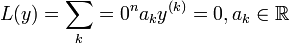$L(y) = \sum_k=0^n a_k y^{(k)} = 0, a_k \in \mathbb{R}$

Failed to parse (syntax error): L:{functions on \mathbb{R} \rightarrow {functions on \mathbb{R}

is a linear transformation ("linear operator").


What do we expect from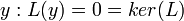${y:L(y) = 0} = ker(L)$? We expect an n-dimensional vector space.

Take Failed to parse (lexing error): y"+y'-6y = 0 , guess Failed to parse (lexing error): y = c, y' = \alpha e^{\alpha x}, y" = \alpha^2e^{\alpha x}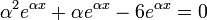$\alpha^2 e^{\alpha x} + \alpha e^{\alpha x} - 6 e^{\alpha x} = 0$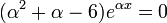$(\alpha^2 +\alpha - 6) e^{\alpha x} = 0$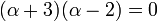$(\alpha +3)(\alpha - 2) = 0$

So we have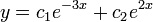$y = c_1e^{-3x} +c_2 e^{2x}$ as the general solution.

Say we have complex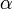$\alpha$. Then what?